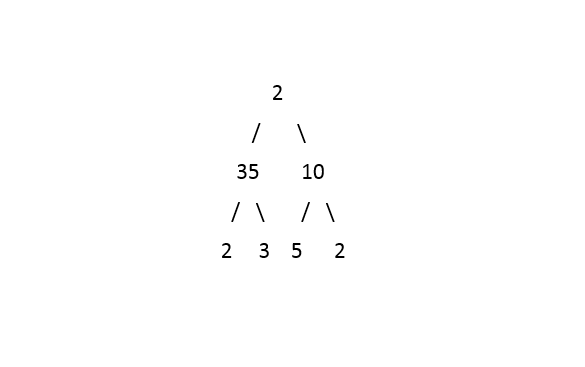# Boundary Sum

Posted: 9 Oct, 2020
Difficulty: Moderate

## PROBLEM STATEMENT

#### Given a Binary Tree of 'N' nodes, find and print the sum of all the boundary nodes. Boundary nodes consist of a root node, leftmost external nodes, rightmost external nodes and all the leaves of the tree.

##### Example :
``````Given binary tree :
````````````Boundary Nodes :
Root : 2
Leftmost : 35
Rightmost : 10
Leaf nodes : 2,3,5,2
Sum : 59
``````
##### Input Format :
``````The first line contains an Integer 'T' which denotes the number of test cases. Then the test cases follow.

The first line of input contains the elements of the tree in the level order form separated by a single space.
If any node does not have left or right child, take -1 in its place. Refer to the example below.

Example :

Elements are in the level order form. The input consists of values of nodes separated by a single space in a single line. In case a node is null, we take -1 on its place.

For example, the input for the tree depicted in the below image would be :
````````````1
2 3
4 -1 5 6
-1 7 -1 -1 -1 -1
-1 -1

Explanation :
Level 1 :
The root node of the tree is 1

Level 2 :
Left child of 1 = 2
Right child of 1 = 3

Level 3 :
Left child of 2 = 4
Right child of 2 = null (-1)
Left child of 3 = 5
Right child of 3 = 6

Level 4 :
Left child of 4 = null (-1)
Right child of 4 = 7
Left child of 5 = null (-1)
Right child of 5 = null (-1)
Left child of 6 = null (-1)
Right child of 6 = null (-1)

Level 5 :
Left child of 7 = null (-1)
Right child of 7 = null (-1)

The first not-null node (of the previous level) is treated as the parent of the first two nodes of the current level. The second not-null node (of the previous level) is treated as the parent node for the next two nodes of the current level and so on.

The input ends when all nodes at the last level are null (-1).
``````
##### Note :
``````The above format was just to provide clarity on how the input is formed for a given tree.

The sequence will be put together in a single line separated by a single space. Hence, for the above-depicted tree, the input will be given as:

1 2 3 4 -1 5 6 -1 7 -1 -1 -1 -1 -1 -1
``````
##### Output Format :
``````For each test case, print a single integer denoting the sum of boundary nodes.

The output of each test case will be printed in a separate line.
``````
##### Note :
``````You do not need to print anything, it has already been taken care of. Just implement the given function.
``````
##### Constraint :
``````1 <= T <= 100
1 <= N <= 1000
-10^6 <= node.data <= 10^6

Where N is the number of nodes in the tree,  and 'node.data' denotes data contained in the node of the binary tree.

Time Limit: 1 sec
``````Approach 1
1. For calculating the boundary sum, we need to calculate 3 things :
• Sum of leftmost nodes
• Sum of rightmost nodes
• Sum of leaf nodes
2. For calculating the sum of leaf nodes we traverse recursively through all the nodes of the binary tree. If we find a node whose left and right child is ‘NULL’ simultaneously then we add that node to the sum.
3. For calculating the sum of the leftmost nodes we start traversing in the left subtree. Firstly, add the current parent node to the sum and check if the left child exists. If the left child exists traverse recursively to the left child node else check for the right child node.
4. If the right child node exists, then traverse recursively to the right child node. Repeat the same until we encounter ‘NULL’.
5. For calculating the sum of the rightmost nodes we start traversing in the right subtree. Firstly, add the current parent node to the sum and check if the right child exists. If the right child exists traverse recursively to the right child node else check for the left child node.
6. If the left child node exists, then traverse recursively to the left child node. Repeat the same until we encounter ‘NULL’.
7. After calculating the sum of leftmost nodes, rightmost nodes, and leaf nodes, add them to get the boundary sum.